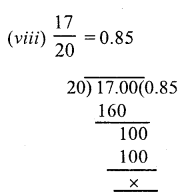# RS Aggarwal Class 7 Solutions Chapter 3 Decimals Ex 3A

In this chapter, we provide RS Aggarwal Solutions for Class 7 Chapter 3 Decimals Ex 3A for English medium students, Which will very helpful for every student in their exams. Students can download the latest RS Aggarwal Solutions for Class 7 Chapter 3 Decimals Ex 3A Maths pdf, free RS Aggarwal Solutions Class 7 Chapter 3 Decimals Ex 3A Maths book pdf download. Now you will get step by step solution to each question.

### RS Aggarwal Solutions for Class 7 Chapter 3 Decimals Ex 3A Download PDF

Question 1.
Solution:Question 2.
Solution:Question 3.
Solution:Question 4.
Solution:
(i) 6.5, 16.03, 0.274, 119.4
In these decimals, the greatest places of decimal is 3
6.5 = 6.500
16.03 = 16.030
0. 274 = 0.274
119.4 = 119.400 are like decimals.
(ii) 3.5, 0.67, 15.6, 4
In these decimal, the greatest place of decimal is 2
3.5 = 3.50
0.67 = 0.67
15.6 = 15.60
4 = 4.00 are the like decimals

Question 5.
Solution:
(i) Among 78.23 and 69.85,
78.23 is greater than 69.85 (78 > 69)
78.23 > 69.85
(ii) Among 3.406 and 3.46,
3.406 is less than 3.46 (40 < 46)
3.406 < 3.46
(iii) Among 5.68 and 5.86,
5.68 is less than 5.86 (68 < 86)
5.68 < 5.86
(iv) Among 14.05 and 14.005
14.5 is greater than 14.005 (05 > 00)
14.5 >14.005
(v) Among 1.85 and 1.805,
1.85 is greater than 1.805 (85 > 80)
1.85 > 1.805
(vi) Among 0.98 and 1.07,
0.98 is less than 1.07 (0 < 1)
0.98 < 1.07

Question 6.
Solution:
(i) 4.6, 7.4, 4.58, 7.32, 4.06
Converting the given decimals into like decimals, we get:
4.60, 7.40, 4.58, 7.32, 4.06.
We see that 4.06 < 4.58 < 4.60 < 7.32 < 7.40.
Writing in ascending order, 4.06, 4.58, 4.6, 7.32, 7.4
(ii) 0.5, 5.5, 5.05, 0.05, 5.55
Converting the given decimals into like decimals, we get:
0. 50, 5.50, 5.05, 0.05, 5.55
We see that 0.05 < 0.50 < 5.05 < 5.50 < 5.55.
Writing in ascending order, 0.05, 0.50, 5.05, 5.5, 5.55
(iii) 6.84, 6.84, 6.8, 6.4, 6.08
Converting the given decimals into like decimals
6.84, 6.48, 6.80, 6.40, 6.08
We see that 6.08 < 6.40 < 6.48 < 6.80 < 6.84
Writing in ascending order,
6.08, 6.4, 6.48, 6.8, 6.84
(iv) 2.2, 2.202, 2.02, 22.2, 2.002
Converting them into like decimals
2.200, 2.202, 2.020, 22.200, 2.002 we see that
2.002 < 2.020 < 2.200 < 2.202 < 22.200
Now writing in ascending order,
2.002, 2.020, 2.2, 2.202, 22.2

Question 7.
Solution:
(i) 7.4, 8.34, 74.4, 7.44, 0.74
Converting them into like decimals,
7.40, 8.34, 74.40, 7.44, 0.74
we see that
74.40 > 8.34 > 7.44 > 7.40 > 0.74
Writing in descending order,
74.4, 8.34, 7.44, 7.4, 0.74
(ii) 2.6, 2.26, 2.06, 2.007, 2.3
Converting them into like decimals,
2.600, 2.260, 2.060, 2.007, 2.300
We see that
2.600 > 2.300 > 2.260 > 2.060 > 2.007
Writing in descending order,
2.6, 2.3, 2.26, 2.06, 2.007

Question 8.
Solution:Question 9.
Solution:Question 10.
Solution:All Chapter RS Aggarwal Solutions For Class 7 Maths

—————————————————————————–

All Subject NCERT Exemplar Problems Solutions For Class 7

All Subject NCERT Solutions For Class 7

*************************************************

I think you got complete solutions for this chapter. If You have any queries regarding this chapter, please comment on the below section our subject teacher will answer you. We tried our best to give complete solutions so you got good marks in your exam.

If these solutions have helped you, you can also share rsaggarwalsolutions.in to your friends.